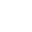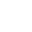# Frustrated that Students Don’t Know The Multiplication Facts?

Have you’ve encountered the student who doesn’t study the multiplication facts? Or the student who learns one multiplication table and then seemingly forgets most of the facts? Or the student who studies but has a hard time recalling the facts?

I’m sure you have!  We introduce multiplication in second grade as counting groups by a certain number (2, 5 and 10).  In third grade, we formally introduce the concept of multiplication through equal groups, arrays, the area model, etc.  And for the most part, most students “get” multiplication as a concept.

But there eventually comes a time when the math will get more complicated (and maybe harder).  So as a mathematician, you want efficiency.  Learning by memory all the multiplication facts will make you a more efficient mathematician when the math does get more complicated.## Should Students Just Memorize the Multiplication Facts?

So now we are back to the students and memorizing the multiplication facts.  There are lots of tips and tricks and strategies for memorizing the multiplication facts.  I’ve even developed resources for my students that give them tips and strategies for remembering the multiplication facts.

But the problem with memory is that for it to be useful, you should be able to recall easily.  So beyond teaching memorization tips and strategies for the multiplication tables, what else can we do?  How can we ensure students learn those facts if they can NOT recall from memory?Teach them strategies for fluency!  Let me show you some mental math strategies that you can teach your students to use with memory is not enough!  But first, what exactly is “fluency?”

## What is Fluency with the Multiplication Facts?

The Common Core DOES specify that memorization of the multiplication tables is an expectation.  But that does not mean that meaningless memorization is the route to go.  We want students to understand multiplication conceptually as well as recalling facts.  In the Common Core, there are various terms used:  know from memory, be fluent in, demonstrate fluency, etc.  It even states that “by the end of Grade 3, know from memory all the products of two one-digit numbers.”

Now, let us clear up some terms.  To know from the memory means to recall from memory a fact.  It is remembering.Fluency is more complicated.  It is about accuracy. It is about speed. It is about recall.  BUT it’s also more than that.  Why, because memory can fail, while the stress of producing or performing can lead to a mental block or shutdown. Fluency is also about using strategies.  Strategies that plug the gap when recalling or “knowing from memory” fails.

## What Are the Strategies to Teach for Multiplication Fluency?

The mental math strategies used to teach multiplication fluency are grouped into two categories:  Foundational Strategies and Derivative Strategies.

The Foundation Strategies are the first strategies to teach and use. They are the mental math strategies of counting by a number (also known as skip counting), knowing the square numbers, and knowing the Zero and Identity Properties of Multiplication.  These are the basic or base multiplication facts.

The Derivative Strategies build on the Foundation Strategies.  They teach halving and doubling, adding or subtracting a group, using a nearby square, the patterns for the nines multiplication table, and the Commutative and Distributive Properties of Multiplication.## Mental Math for the Multiplication Facts

The strategies are similar in use to the addition strategies you would teach students learning to add.  In addition, they include decomposing a number, doubles, doubles plus one, adding one more, etc.  These are mental math strategies that are explicitly taught and practiced with pencil and paper first. After that, they become part of the mental math repertoire for adding.

The same applies to these multiplication strategies.  They are explicitly taught and practiced with pencil and paper until the student can use the strategy in mental math.Are you eager to see the rest?  Then download the FREE Guide to Achieving Multiplication Fluency!

It’s a 15-page guide that explains all the strategies.  It has some suggestions for teaching these strategies and how to assess strategy use.  Get your students on the road to fluency with the multiplication facts!

It’s FREE and you can download by subscribing to my newsletter. Click HERE or fill out the form below

## Don’t forget to read Parts 2 and 3 of This 3 Part Series!

In Part 2 of this 3 part blog series, come back to learn more about how to teach the Foundation Strategies.

In Part 3, we’ll take a look at the Derivative Strategies.

Here’s the link to PART 2:  Foundation Strategies.

Here’s the link to PART 3:  Derivative Strategies

In the meantime, check out the latest resource in my Teachers Pay Teachers store!  Made explicitly for teaching multiplication fluency strategies!## Looking for More Multiplication Ideas, Tips, and Lessons? Check out the articles below!

Distributive Property of Multiplication – How to Break it Down

Learning Multiplication is More than Memorization!

Multiplication Practice Made Fun and Easy

## Don’t Go Yet!

Are you new to the 1:1 classroom setting? Then you’ll want to read my Valuable Tips for the 1:1 Classroom.

Check out how I use Google Classroom to present at Back to School Night for Parents.

Or register for the newsletter to receive this FREE Guide to Achieving Multiplication Fluency. Get it now by signing up for my newsletter below!###### Follow:

I’m a single dad raising two boys, 15 and 12. I’m also a veteran teacher of over 30 years who has taught first, second and third grades. I’m also a trained literacy coach, served as a mentor teacher and have a Bilingual Specialist Credential. I’m currently serving as a District Math Coach.

Share:

### 1 Comment

1.January 11, 2020 / 6:35 pm

What strategies are you using for multiplication fluency? Do your students rely on just memorization? Are they using known facts? Properties of Multiplication? Share your ideas!

### Interactive Digital Notebooks for Google Slides®